# Gentlest Introduction to Tensorflow #2

Summary: We show in illustrations how the machine learning ‘training’ process happens in Tensorflow, and tie them back to the Tensorflow code. This paves the way for discussing ‘training’ variations, namely stochastic/mini-batch/batch, and adaptive learning rate gradient descent. The ‘training’ variation code snippets presented serve to reinforce the understanding of the role of Tensorflow placeholders.

This is part of a series:

• Part 1: Linear regression with Tensorflow for single feature single outcome model
• Part 2 (this article): Tensorflow training illustrated in diagrams/code, and exploring training variations
• Part 3: Matrices and multi-feature linear regression with Tensorflow
• Part 4: Logistic regression with Tensorflow

### Quick Review

In the previous article, we used Tensorflow (TF) to build and learn a linear regression model with a single feature so that given a feature value (house size/sqm), we can predict the outcome (house price/\$).

Here is the review with illustration below:

1. We have some data of house sizes & house prices (the gray round points)
2. We model the data using linear regression (the red dash line)
3. We find the ‘best’ model by training W, and b (of the linear regression model) to minimize the ‘cost’ (the sum of the length of vertical blue lines, which represent the differences between predictions and actual outcomes)
4. Given any house size, we can use the linear model to predict the house size (the dotted blue lines with arrows)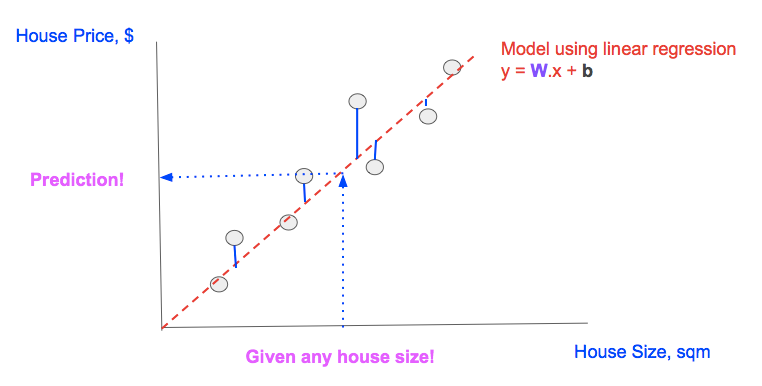Linear regression explained in a single diagram

In machine learning (ML) literature, we come across the term ‘training’ very often, let us literally look at what that means in TF.

### Linear Regression Modeling

`Linear Model (in TF notation): y = tf.matmul(x,W) + b`

The goal in linear regression is to find W, b, such that given any feature value (x), we can find the prediction (y) by substituting W, x, b values into the model.

However to find W, b that can give accurate predictions, we need to ‘train’ the model using available data (the multiple pairs of actual feature (x), and actual outcome (y_), note the underscore).

### ‘Training’ Illustrated

To find the best W, b values, we can initially start with any W, b values. We also need to define a cost function, which is a measure of the difference between the prediction (y) for given a feature value (x), and the actual outcome (y_) for that same feature value (x). For simplicity, we use least minimum squared error (MSE) as our cost function.

`Cost function (in TF notation): tf.reduce_mean(tf.square(y_ - y))`

By minimizing the cost function, we can arrive at good W, b values.

Our code to do training is actually very simple and it is labelled with [A, B, C, D], which we will refer to later on. The full source is on Github.

`# ... (snip) Variable/Constants declarations (snip) ...`
`# [A] TF.Graphy = tf.matmul(x,W) + bcost = tf.reduce_mean(tf.square(y_-y))`
`# [B] Train with fixed 'learn_rate'learn_rate = 0.1train_step =    tf.train.GradientDescentOptimizer(learn_rate).minimize(cost)`
`for i in range(steps):  # [C] Prepare datapoints  # ... (snip) Code to prepare datapoint as xs, and ys (snip) ...`
`  # [D] Feed Data at each step/epoch into 'train_step'  feed = { x: xs, y_: ys }  sess.run(train_step, feed_dict=feed)`

Our linear model and cost function equations [A] can be represented as TF graph as shown: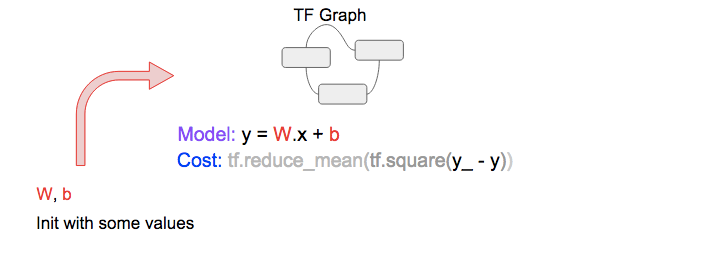Create a TF Graph with model & cost, and initialize W, b with some values

Next, we select a datapoint (x, y_) [C], and feed [D] it into the TF Graph to get the prediction (y) as well as the cost.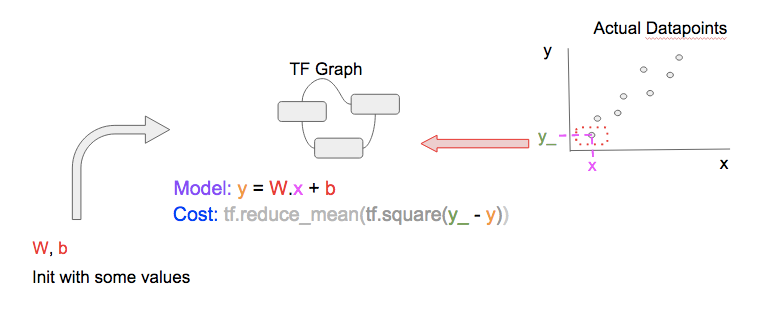Calculate prediction (y) & cost using a single datapoint

To get better W, b, we perform gradient descent using TF’s tf.train.GradientDescentOptimizer [B] to reduce the cost. In non-technical terms: given the current cost, and based on the graph of how cost varies with other variables (namely W, b), the optimizer will perform small tweaks (increments/decrements) to W, b so that our prediction becomes better for that single datapoint.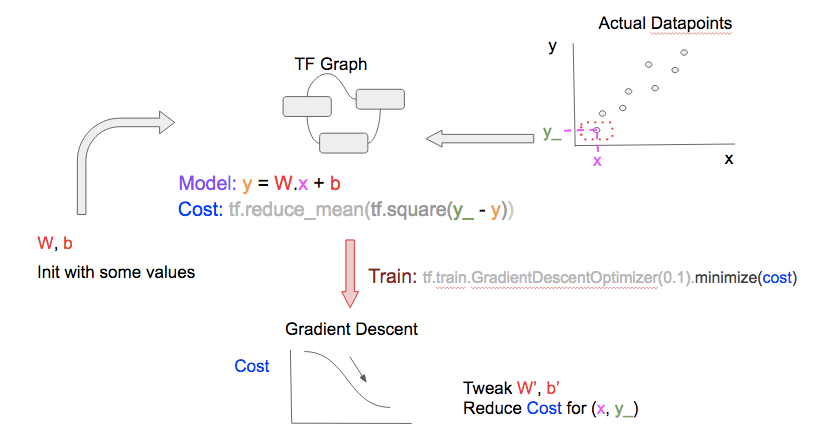Based on current cost, determine how to tweak W, b to improve prediction (y) and reduce cost

The final step in the training cycle is to update the W, b after tweaking them. Note that ‘cycle’ is also referred to as ‘epoch’ in ML literature.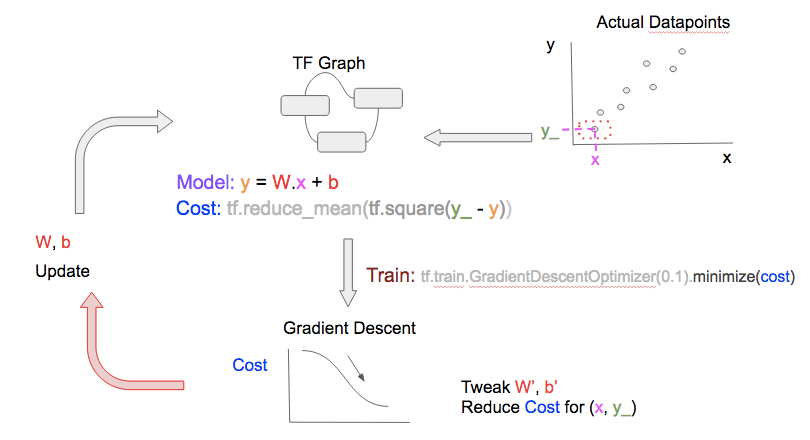Update W, b after tweaking them, and before iterating through the next training epoch

In the next training epoch, repeat the steps, but use a different datapoint!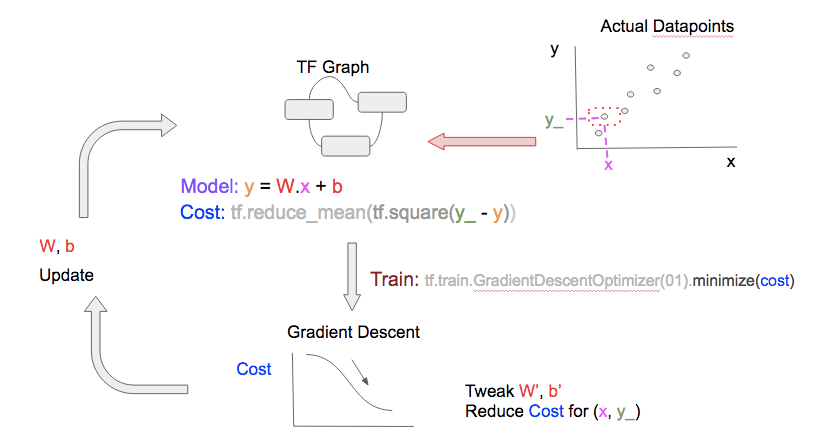Training using different datapoints

Using a variety of datapoints generalizes our model, i.e., it learns W, b values that can be used to predict any feature value. Note that:

• In most cases, the more datapoints, the better your model can learn and generalize
• If you train more epochs than datapoints you have, you can re-use datapoints, which is not an issue. The gradient descent optimizer always use both the datapoint, AND the cost (calculated from the datapoint, with W, b values of that epoch) to tweak W, b; the optimizer may have seen that datapoint before, but not with the same cost, thus it will learn something new, and tweak W, b differently.

You can train the model a fixed number of epochs or until it reaches a cost threshold that is satisfactory.

### Training Variation

#### Stochastic, Mini-batch, Batch

In the training above, we feed a single datapoint at each epoch. This is known as stochastic gradient descent. We can feed a bunch of datapoints at each epoch, which is known as mini-batch gradient descent, or even feed all the datapoints at each epoch, known as batch gradient descent. See the graphical comparison below and note the 2 differences between the 3 diagrams:

• The number of datapoints (upper-right of each diagram) fed to TF.Graph at each epoch
• The number of datapoints for the gradient descent optimizer to consider when tweaking W, b to reduce cost (bottom-right of each diagram)Stochastic gradient descent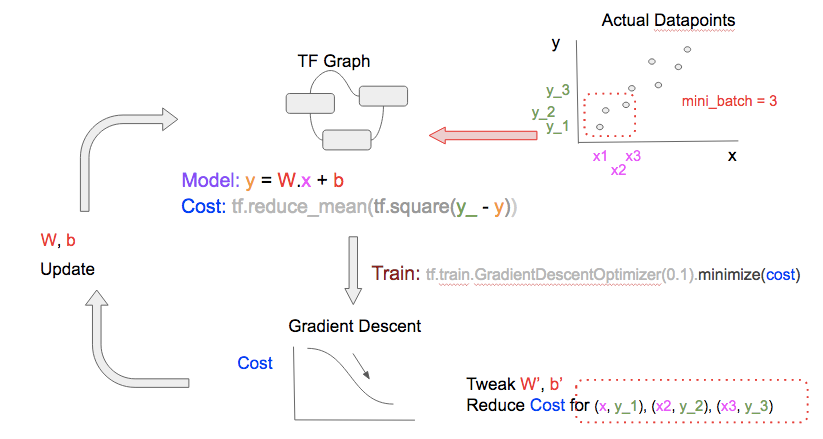Mini-batch gradient descent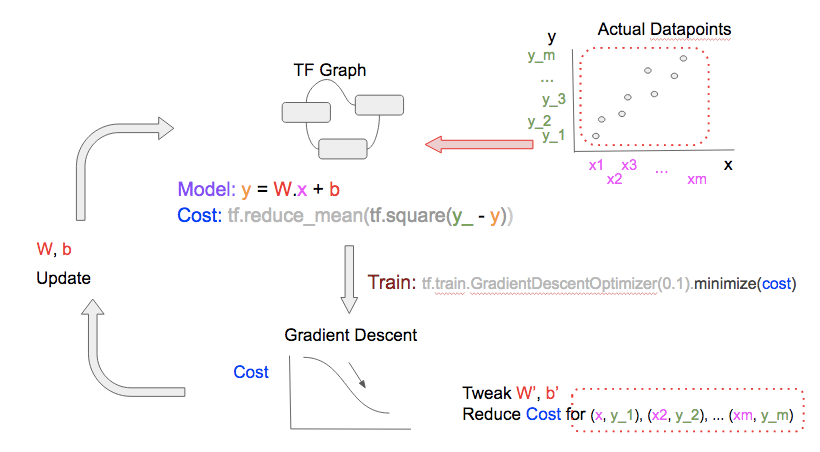Batch gradient descent

The number of datapoints used at each epoch has 2 implications. With more datapoints:

• Computational resource (subtractions, squares, and additions) needed to calculate the cost and perform gradient descent increases
• Speed at which the model can learn and generalize increases

The pros and cons of doing stochastic, mini-batch, batch gradient descent can be summarized in the diagram below: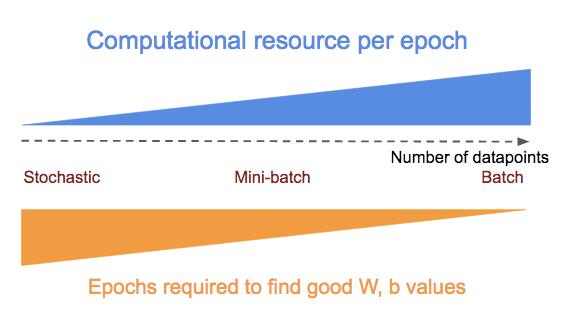Pros and cons of stochastic, mini-batch & batch gradient descent

To switch between stochastic/mini-batch/batch gradient descent, we just need to prepare the datapoints into different batch sizes before feeding them into the training step [D], i.e., use the snippet below for[C]:

`# * all_xs: All the feature values# * all_ys: All the outcome values# datapoint_size: Number of points/entries in all_xs/all_ys# batch_size: Configure this to:#             1: stochastic mode#             integer < datapoint_size: mini-batch mode#             datapoint_size: batch mode# i: Current epoch number`
`if datapoint_size == batch_size:  # Batch mode so select all points starting from index 0  batch_start_idx = 0elif datapoint_size < batch_size:  # Not possible  raise ValueError(“datapoint_size: %d, must be greater than                             batch_size: %d” % (datapoint_size, batch_size))else:  # stochastic/mini-batch mode: Select datapoints in batches  #                             from all possible datapoints  batch_start_idx = (i * batch_size) % (datapoint_size — batch_size)  batch_end_idx = batch_start_idx + batch_size  batch_xs = all_xs[batch_start_idx:batch_end_idx]  batch_ys = all_ys[batch_start_idx:batch_end_idx]`
`# Get batched datapoints into xs, ys, which is fed into# 'train_step'xs = np.array(batch_xs)ys = np.array(batch_ys)`

#### Learn Rate Variation

Learn rate is how big an increment/decrement we want gradient descent to tweak W, b, once it decides whether to increment/decrement them. With a small learn rate, we will proceed slowly but surely towards minimal cost, but with a larger learn rate, we can reach the minimal cost faster, but at the risk of ‘overshooting’, and never finding it.

To overcome this, many ML practitioners use a large learn rate initially (with the assumption that initial cost is far away from minimum), and then decrease the learn rate gradually after each epoch.

TF provides 2 ways to do so as wonderfully explained in this StackOverflow thread, but here is the summary.

Use Gradient Descent Optimizer Variants

TF comes with various gradient descent optimizer, which supports learn rate variation, such as tf.train.AdagradientOptimizer, and tf.train.AdamOptimizer.

Use tf.placeholder for Learn Rate

As you have learned previously, if we declare a tf.placeholder, in this case for learn rate, and use it within the tf.train.GradientDescentOptimizer, we can feed a different value to it at each training epoch, much like how we feed different datapoints to x, y_, which are also tf.placeholders, at each epoch.

We need 2 slight modifications:

`# Modify [B] to make 'learn_rate' a 'tf.placeholder'# and supply it to the 'learning_rate' parameter name of# tf.train.GradientDescentOptimizerlearn_rate = tf.placeholder(tf.float32, shape=[])train_step = tf.train.GradientDescentOptimizer(    learning_rate=learn_rate).minimize(cost)`
`# Modify [D] to include feed a 'learn_rate' value,# which is the 'initial_learn_rate' divided by# 'i' (current epoch number)# NOTE: Oversimplified. For example only.feed = { x: xs, y_: ys, learn_rate: initial_learn_rate/i }sess.run(train_step, feed_dict=feed)`

### Wrapping Up

We illustrated what machine learning ‘training’ is, and how to perform it using Tensorflow with just model & cost definitions, and looping through the training step, which feeds datapoints into the gradient descent optimizer. We also discussed the common variations in training, namely changing the size of datapoints the model uses for learning at each epoch, and varying the learn rate of gradient descent optimizer.

#### Coming Up Next

• Set up Tensor Board to visualize TF execution to detect problems in our model, cost function, or gradient descent
• Perform linear regression with multiple features